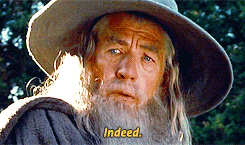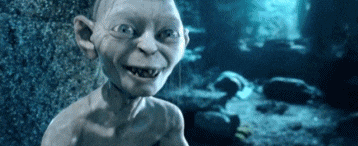# Graph - Data Structure & Algorithm Part VIII

Hello everyone, today I intend to continue this series that we discussed data structure and for this post we will learn graphs. This incredible structure you can apply to many problems in the real world, so it is one of the most important structures and also very common in interview questions.

So, let's dive into this new adventure!

💭 "The trick is to fix the problem you have, rather than the problem you want." - Bram Cohen

### Outline

• What is a Graph?
• Basic concepts.
• The main operations and properties.
• Learning BFS and DFS functions.

### Graph

Many people often confuse a graph with a tree structure, and that's happened because a tree is a type of graph!Basically, a graph is a non-linear structure of a set of vertices V connected by edges E that can be represented as ordered pair of vertices G(V,E) .

More precisely, a graph is composed of paths that contain adjacency vertices connected by edges. Usually, you can find many books and articles using different terms to refer to vertices and edges, the most common of which are:

• Vertex: Nodes or points;
• Edges: Lines, links or arcs;

### ▪️ Graph visualization

One of the most interesting things that make graphs a powerful structure is how they can represent a lot of information for some applications. There are many examples that we can use and the most common are a network of cities, streets, flights, ferries, railway maps, social network connections, and so on...

From theses examples, a graph structure can obtain a lot of information, such as how many cities are close to another or which is the sort path between two cities, for example. Can you see how powerful this structure can be?

Even though a graph is just a collection of nodes and edges, there are two ways to represent it, which are:

As the name suggests, this representation uses a square matrix where rows and columns mean that there is a relationship from one vertex to another. We can see how it works in the image below.###### Figure 1: Adjacency Matrice visualization.

As we can see, the matrix represents which vertices are connected by edges, we can simply find out if there is a relationship between the vertices looking at the matrix.

Is the most common and efficient way to represent a graph, because creates an array that can store all vertices in a list for each vertex of the graph. Using the same set used in the adjacency matrix above:### ▪️ Graph Representations

After talking about visualizations, the next step is to learn how many types of a graph exists. Here we will see how the vertices are organized and connected.

### Directed or undirected

• Directed

In this type of graph, the edges are directed from one vertex to another. As we can see, the edge between 0 and 1 vertices is directed, right?###### Figure 3: Directed graph representation.
• Undirected

Unlike the directed graph, this type of graph has all vertices pointing towards each other, that is, all edges are bidirectional.###### Figure 4: Undirected graph representation.
• Cyclic or acyclic

A cycle graph means if the graph contains a path that begins at a given vertex and after few vertices ends at the same starting vertex. The example below contains the following cycle: 5 -> 2 -> 1 -> 4.### ▪️ Basic operations

Okay, now that we already understand what a graph is, let’s see how to implement it. First thing first, we need to create our main class and, as we have seen, there are two ways to build this structure and will use the adjacency list representation, where a key and all its connections are associated.

Let's see how below:

``````class Graph {
constructor() {
}
...

}
``````

Simple right? 😁 We just need to initialize the adjacencyList variable that will be used as a dictionary to add key-value pairs. The next step is to know how to insert a vertex in our graph using the dictionary that was created.

When a new vertex is added to the structure, it takes only a constant time, the time complexity of O(1). This is because we just need to add the new element to the array.

Great! Moving forward, we need to use a real-world example to facilitate our understanding and we will use a social network to exemplify operations.

``````addVertex(vertex){
}
``````

A social network needs some users, right? So, let's fill it out by adding some people from middle-earth using the following code:

``````const middle_earth = new Graph();

``````

Well, something is missing from our social network. We need interactions between the users, the next step is to create some connections between the vertices created.

As discussed earlier, the main differences between these types of graphs are in the fact that only the undirected function creates connections on both sides.

The code below shows how we can create connections using directed and undirected approaches.

``````addEdgeDirected(vertex1, vertex2) {
}
}

}

}
}

}
``````

In this example of social networking, we will use the undirected approach, however, the directed type graph also can be used. Moving on, let's now imagine that Gandalf added some hobbits and a wizard to his social network.

``````middle_earth.addEdgeUndirected('Gandalf', 'Billy');
``````

After that, our graph looks something like this:###### Figure 6: Middle-earth network representation.

Awesome! 😁

Okay, moving forward with the discussion, let's imagine the scenario where the Sméagol user had a bad behaviour and it is necessary to removed it, how we can do this?For now, we have no way to remove any vertex. So, we need to fix this, right? To delete a vertex from the graph, it is necessary to iterate through the list of each vertex and validate whether an edge exists or not. If it exists, then we have to remove the vertex. Let’s take a look:

``````removeVertex(vertex) {
vertexList.forEach(element => {
}
});
}
}
``````

As you may be thinking, this function is O(n) because we need to iterate over the vertices and then remove the element.

And the edges? I mean, what if Gandalf had a big argument with Saruman and then decides to remove him from his social network? What do we have to do? 🧐 Well, to remove an edge, we need to check if the vertices are connected and then remove them from the array.

``````removeEdge(vertex1, vertex2) {
}
}
``````

Great! Can you see the progress? 🚀

There are functions like print_all_path_destination, print_adjacency_matrix, countPaths, isAdjacent and others that I would like to discuss, but I’ll skip the explanations for not taking too much of your time.

⚡️ But if you would like to learn or see others functions that I implemented you can access all the code just clicking here.

Now we are going to use all the acknowledgement that we learned to implement search function.

### ▪️ Graph Search

Let's dive into the most important topic related to this structure! We want to traverse all the vertices and edges of that graph. What does that mean? Well, we can put an example: Gandalf had a map and try to travel across middle-earth. 😁 But relax, we'll see all the steps of these functions using an example along the way. Let's create a graph to be used.

``````var graph_example = new Graph();
``````

After creating the vertices and edges, our graph will look something like this:###### Figure 7: Graph example to be used on BFS and DFS functions.

This approach is the most common and used. It starts by considering all vertices as unvisited and all edges undiscovered. With that in mind, we can choose an arbitrary vertex and then discover all vertices connected by an edge and visited.

Every time that an adjacent vertex is visited, we must mark it and insert it in a queue. Since none of the edges that incident on the vertex are undiscovered, we can proceed and explore the next vertex.

Using the example above and considering vertex 0 as the current vertex, the result is:

``````Visited Vertex: 0
Visited Vertex: 1
Visited Vertex: 2
Visited Vertex: 3
Visited Vertex: 4
Visited Vertex: 5
Visited Vertex: 6
``````

We must repeat the process until no undiscovered and unvisited are left in the structure. When the queue is empty, it means that the algorithm covers all vertices and edges. With all that in mind, let's put everything in a code.

``````breadthFirstSearch(current_vertice) {
if(vertices.length === 0){
return;
}else {
var discovered = {};
vertices.forEach(function(item) {
discovered[item] = false;
})
}
}

var queue = [];
discovered[vertex] = true;
queue.push(vertex);

while(queue.length > 0){
var u = queue.shift();
console.log('Visited Vertex: ' + u);

for (let index = 0; index < listAdjacents.length; index++) {
if(!discovered[element]){
discovered[element] = true;
queue.push(element);
}
}
}
}
``````
• Depth First Search (DFS)

Initially, this function has conditions similar to the BFS function, all vertices are unvisited and edges are not discovered. Then, we can choose an arbitrary vertex that will be our root element, which will be visited and called the current vertex.

Now is when the difference between DFS and BFS functions begins! The current vertex has to explore as far as possible along each vertex visited, moving to the next undiscovered adjacent edge and printing the path.

We must continue this loop until there are no unvisited and undiscovered elements. Instead of queuing, the DFS function uses a stack to find the shortest path. After that, with no undiscovered edges left, we have to go back to the initial visited vertex and start again checking other unvisited vertices until cover all vertices and edges of the graph.

Using vertex 0 as the current vertex, we will obtain the following result:

``````Visited Vertex  0
Visited Vertex  1
Visited Vertex  3
Visited Vertex  4
Visited Vertex  2
Visited Vertex  5
Visited Vertex  6
``````
``````depthFirstSearch(current_vertice) {
if(vertices.length === 0){
return;
}
var discovered = {};
vertices.forEach(function(item) {
discovered[item] = false;
})
this._depthFirstSearch(current_vertice, discovered);
}

_depthFirstSearch(current_vertice, discovered){
discovered[current_vertice] = true;
console.log('Visited Vertex ', current_vertice);

for (let index = 0; index < listAdjacents.length; index++) {
if(!discovered[element]){
this._depthFirstSearch(element, discovered);
}
}
}
``````

That's all folks!

I hope you have fun learning. 😁So we finished our discussion about Graph structure. 🙌

If you found something I miss out or find this article helpful, feel free to let me know. 😁